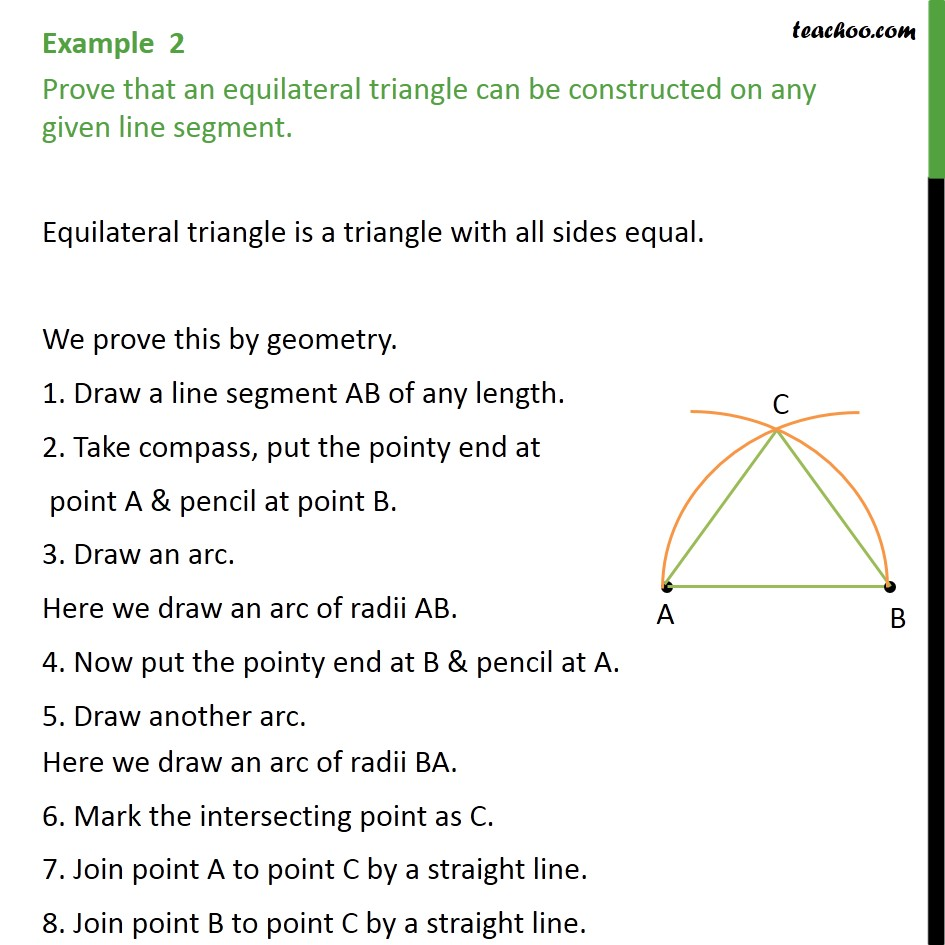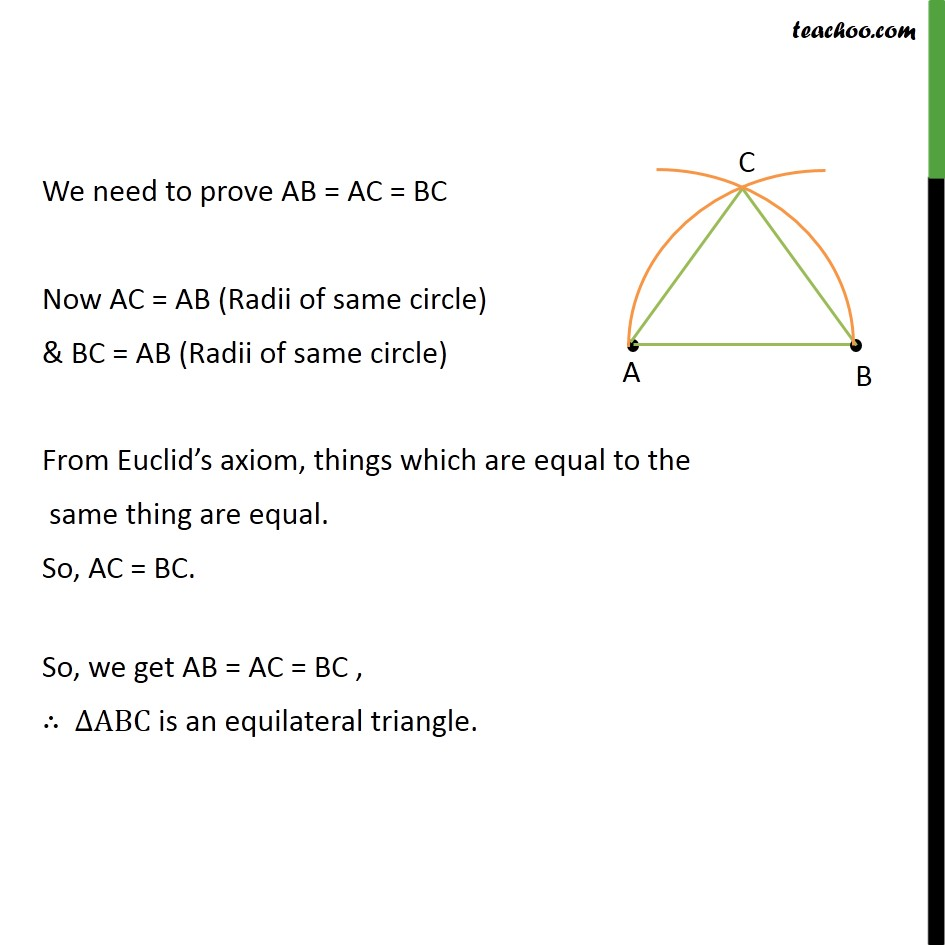Examples

Chapter 5 Class 9 Introduction to Euclid's Geometry
Serial order wiseLearn in your speed, with individual attention - Teachoo Maths 1-on-1 Class

### Transcript

Example 2 Prove that an equilateral triangle can be constructed on any given line segment. Equilateral triangle is a triangle with all sides equal. We prove this by geometry. 1. Draw a line segment AB of any length. 2. Take compass, put the pointy end at point A & pencil at point B. 3. Draw an arc. Here we draw an arc of radii AB. 4. Now put the pointy end at B & pencil at A. 5. Draw another arc. Here we draw an arc of radii BA. 6. Mark the intersecting point as C. 7. Join point A to point C by a straight line. 8. Join point B to point C by a straight line. We need to prove AB = AC = BC Now AC = AB (Radii of same circle) & BC = AB (Radii of same circle) From Euclid’s axiom, things which are equal to the same thing are equal. So, AC = BC. So, we get AB = AC = BC , ∴ ∆ABC is an equilateral triangle.